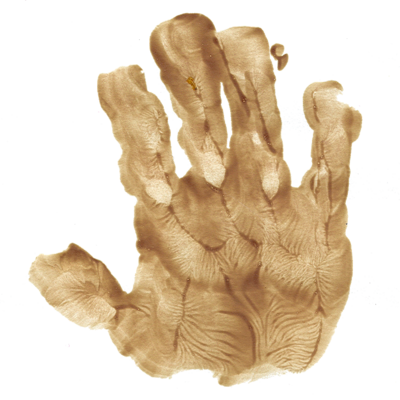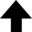Since a given system can never of its own accord go over into another equally probable state but into a more probable one, it is likewise impossible to construct a system of bodies that after traversing various states returns periodically to its original state.Ludwig Boltzmann

For an ideal gas, the energy of a configuration is independent of the positions of the particles, and the total energy of the system is the sum of the kinetic energies of the individual particles. Since kinetic energy is proportional to the square of the velocity, for an ideal gas the only coordinates of interest are the velocity coordinates. To change a configuration, we simply choose a particle at random and change its velocity by a small but random amount.

This program implements a microcanonical Monte Carlo simulation of a classical ideal gas in one dimension. The variable MCS, the number of Monte Carlo steps per particle, plays an important role in Monte Carlo simulations. The output of K_avg and v_avg—the mean kinetic energy and mean velocity of the gas, respectively—is in arbitrary units.

## Monte Carlo Simulation of an Ideal Gas

This program investigates some of the equilibrium properties of a one-dimensional classical ideal gas.
 Number of Monte Carlo steps: Mean KE: Mean velocity: Number of rejected Monte Carlo steps:
0%